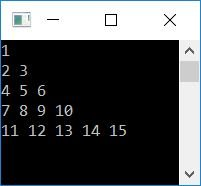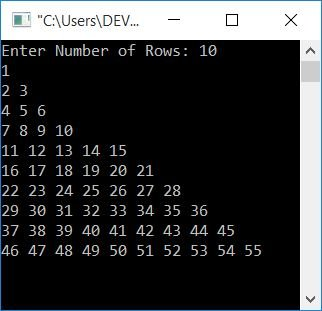# C Program to Print Floyd's Triangle

This article will teach you how to print Floyd's triangle in C and provide you with code to do so. But before going through the program, let's first understand Floyd's Triangle.

### What is Floyd's Triangle?

Floyd's triangle is a right-angled triangle created using natural numbers, as shown here:

```1
2 3
4 5 6
7 8 9 10
11 12 13 14 15```

may continue up to any number of rows. Here, Floyd's triangle is shown up to 5 rows only.

## Print Floyd's Triangle in C

Now let's create a program to print Floyd's triangle with 5 lines or rows:

```#include<stdio.h>
#include<conio.h>
int main()
{
int i, j, num=1;
for(i=0; i<5; i++)
{
for(j=0; j<=i; j++)
{
printf("%d ", num);
num++;
}
printf("\n");
}
getch();
return 0;
}```

This program was built and runs in the Code::Blocks IDE. Here is its sample run:The dry run of the above program goes like this:

• Initially num=1
• Inside the first (outer) for loop, 0 is initialized to i, and it is checked whether it is less than 5 or not.
• The condition is true. Therefore, program flow goes inside the loop.
• Now inside the second for loop, 0 is initialized to j and it is checked whether it is less than or equal to the value of i (0) or not.
• The condition is true. Therefore, program flow goes inside the loop and prints the value of num, which is 1, and then increments its value.
• Now num=2
• The program flow goes to the update part of the inner for loop and increments the value of j. Now the value of j is 2.
• Program flow goes to the condition part and checks whether the value of j is less than or equal to the value of i or not.
• The condition is evaluated as false. Therefore, program flow exits from the inner for loop and prints a new line character. That is, the next output's things begin on the next line.
• Now program flow goes to update part of the outer for loop. There, it increments the value of i and checks whether it is less than 5 or not.
• The condition evaluates to true once more. Therefore, program flow again goes inside the loop and executes the inner loop again.
• Continue the process until the condition of the outer for loop evaluates to false.

### Allow the user to define the size of Floyd's triangle

Here is another program that does the same job as the previous one, which is to print Floyd's triangle. But the user is allowed to define the size of Floyd's triangle. In other words, the program asks the user how many lines Floyd's triangle should expand to.

```#include<stdio.h>
#include<conio.h>
int main()
{
int i, j, num=1, row;
printf("Enter Number of Rows: ");
scanf("%d", &row);
for(i=0; i<row; i++)
{
for(j=0; j<=i; j++)
{
printf("%d ", num);
num++;
}
printf("\n");
}
getch();
return 0;
}```

Here is its sample run, supposing the user input is 10:#### The same program in different languages

C Quiz

« Previous Program Next Program »